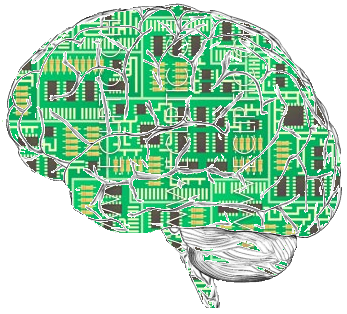Explore BrainMass

# Theory of Computation[ Photo credit: Gengiskanhg]

The theory of computation is primarily centered around how efficiently and completely and algorithm can be used to solve a problem. The three main branches within the theory of computation are:

• Automata theory - the idea that automating processes is the way forward in problem solving. Commonly represented in using an infinite formal language, it aims to recognize and accept any subset of that language as efficiently as possible.
• Computability theory - this branch looks more at the practical use of a computer to problem-solve. It's growth as a sub-field of computational theory was largely kickstarted by the discovery of the impossibility of solving the halting problem on a Turing machine.
• Computational complexity theory - generally the most 'important' of the three branches, this sub-field of computational theory handles the complexities of algorithms in terms of the time and space they take.

There are many famous problems in computational theory, below are just some of them:

• the Halting problem - can a computer determine for certain if a program will run to completion or terminate with an error?
• P vs NP - can every problem whose solution may be quickly verified by a computer also be solved by one?
• The fastest algorithm for matrix multiplication - we have a very quick one now but it is believed there can still be improvements
• The existence or non-existence of one-way functions - are there functions that are easy to compute given input but hard to invert when given the image of random input?